# Factorization of polynomials

(diff) ← Older revision | Latest revision (diff) | Newer revision → (diff)

factoring polynomials

Since C.F. Gauss it is known that an arbitrary polynomial over a field or over the integers can be factored into irreducible factors, essentially uniquely (cf. also Factorial ring). For an efficient version of Gauss' theorem, one asks to find these factors algorithmically, and to devise such algorithms with low cost.

Based on a precocious uncomputability result in [a13], one can construct sufficiently bizarre (but still "computable" ) fields over which even square-freeness of polynomials is undecidable in the sense of A.M. Turing (cf. also Undecidability; Turing machine). But for the fields of practical interest, there are algorithms that perform extremely well, both in theory and practice. Of course, factorization of integers and thus also in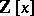remains difficult; much of cryptography (cf. Cryptology) is based on the belief that it will remain so. The base case concerns factoring univariate polynomials over a finite fieldwithelements, whereis a prime power. A first step is to make the input polynomial, of degree, square-free. This is easy to do by computing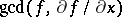and possibly extractingth roots, where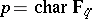. The main tool of all algorithms is the Frobenius automorphism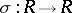on the-algebra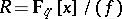. The pioneering algorithms are due to E.R. Berlekamp [a1], [a2], who representsby its matrix on the basis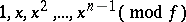of. A second approach, due to D.G. Cantor and H. Zassenhaus [a3], is to computeby repeated squaring. A third method (J. von zur Gathen and V. Shoup [a8]) uses the so-called polynomial representation ofas its basic tool. The last two algorithms are based on Gauss' theorem thatis the product of all monic irreducible polynomials in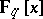whose degree divides. Thus,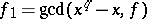is the product of all linear factors of; next,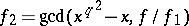consists of all quadratic factors, and so on. This yields the distinct-degree factorization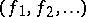of.

The equal-degree factorization step splits any of the resulting factors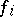. This is only necessary if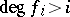. Since all irreducible factors ofhave degree, the algebra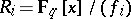is a direct product of (at least two) copies of. A random elementof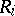is likely to have Legendre symbolin some and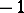in other copies; thenis a non-trivial factor of. To describe the cost of these methods, one uses fast arithmetic, so that polynomials overof degree up tocan be multiplied withoperations in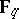, or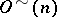for short, where the so-called "soft O" hides factors that are logarithmic in. Furthermore,is an exponent for matrix multiplication, with the current (in 2000) world record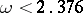, from [a4].

All algorithms first computemodulo, with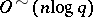operations in. One can show that in an appropriate model,operations are necessary, even for. The further cost is as follows:'

<tbody> </tbody>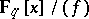as Cost in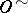Berlekamp-vector space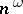Cantor–Zassenhaus multiplicative semi-group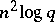von zur Gathen–Shoup-algebraFor small fields, even better algorithms exist.

The next problem is factorization of some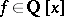. The central tool is Hensel lifting, which lifts a factorization ofmodulo an appropriate prime numberto one modulo a large power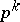of. Irreducible factors ofwill usually factor modulo, according to the Chebotarev density theorem. One can then try various factor combinations of the irreducible factors moduloto recover a true factor in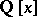. This works quite well in practice, at least for polynomials of moderate degree, but uses exponential time on some inputs (for example, on the Swinnerton-Dyer polynomials). In a celebrated paper, A.K. Lenstra, H.W. Lenstra, Jr. and L. Lovász [a12] introduced basis reduction of integer lattices (cf. LLL basis reduction method), and applied this to obtain a polynomial-time algorithm. Their reduction method has since found many applications, notably in cryptanalysis (cf. also Cryptology). A method in [a10] promises an even faster factorizing method.

The next tasks are bivariate polynomials. It can be solved in a similar fashion, with Hensel lifting, say, modulo one variable, and an appropriate version of basis reduction, which is easy in this case. Algebraic extensions of the ground field are handled similarly.

Multivariate polynomials pose a new type of problem: how to represent them? The dense representation, where each term up to the degree is written out, is often too long. One would like to work with the sparse representation, using only the non-zero coefficients. The methods discussed above can be adapted and work reasonably well on many examples, but no guarantees of polynomial time are given. Two new ingredients are required. The first are efficient versions (due to E. Kaltofen and von zur Gathen) of Hilbert's irreducibility theorem (cf. also Hilbert theorem). These versions say that if one reduces many to two variables with a certain type of random linear substitution, then each irreducible factor is very likely to remain irreducible. The second ingredient is an even more concise representation, namely by a black box which returns the polynomial's value at any requested point. A highlight of this theory is the random polynomial-time factorization method in [a11].

Each major computer algebra system has some variant of these methods implemented. Special-purpose software can factor huge polynomials, for example of degree more than one million over. Several textbooks describe the details of some of these methods, e.g. [a9], [a5], [a6], [a14].

Factorization of polynomials modulo a composite number presents some surprises, such as the possibility of exponentially many irreducible factors, which can nevertheless be determined in polynomial time, in an appropriate data structure; see [a7].

For a historical perspective, note that the basic idea of equal-degree factorization was known to A.M. Legendre, while Gauss had found, around 1798, the distinct-degree factorization algorithm and Hensel lifting. They were to form part of the eighth chapter of his "Disquisitiones Arithmeticae" , but only seven got published, due to lack of funding.

 [a1] E.R. Berlekamp, "Factoring polynomials over finite fields" Bell Syst. Techn. J. , 46 (1967) pp. 1853–1859 [a2] E.R. Berlekamp, "Factoring polynomials over large finite fields" Math. Comput. , 24 : 11 (1970) pp. 713–735 [a3] D.G. Cantor, H. Zassenhaus, "A new algorithm for factoring polynomials over finite fields" Math. Comput. , 36 : 154 (1981) pp. 587–592 [a4] D. Coppersmith, S. Winograd, "Matrix multiplication via arithmetic progressions" J. Symbolic Comput. , 9 (1990) pp. 251–280 [a5] I.E. Shparlinski, "Finite fields: theory and computation" , Kluwer Acad. Publ. (1999) [a6] J. von zur Gathen, J. Gerhard, "Modern computer algebra" , Cambridge Univ. Press (1999) [a7] J. von zur Gathen, S. Hartlieb, "Factoring modular polynomials" J. Symbolic Comput. , 26 : 5 (1998) pp. 583–606 [a8] J. von zur Gathen, V. Shoup, "Computing Frobenius maps and factoring polynomials" Comput. Complexity , 2 (1992) pp. 187–224 [a9] K.O. Geddes, S.R. Czapor, G. Labahn, "Algorithms for computer algebra" , Kluwer Acad. Publ. (1992) [a10] M. van Hoeij, "Factoring polynomials and the knapsack problem" www.math.fsu.edu/~hoeij/knapsack/paper/knapsack.ps (2000) [a11] E. Kaltofen, B.M. Trager, "Computing with polynomials given by black boxes for their evaluations: Greatest common divisors, factorization, separation of numerators and denominators" J. Symbolic Comput. , 9 (1990) pp. 301–320 [a12] A.K. Lenstra, H.W. Lenstra, Jr., L. Lovász, "Factoring polynomials with rational coefficients" Math. Ann. , 261 (1982) pp. 515–534 [a13] B.L. van der Waerden, "Eine Bemerkung über die Unzerlegbarkeit von Polynomen" Math. Ann. , 102 (1930) pp. 738–739 [a14] Chee Keng Yap, "Fundamental problems of algorithmic algebra" , Oxford Univ. Press (2000)
How to Cite This Entry:
Factorization of polynomials. Encyclopedia of Mathematics. URL: http://encyclopediaofmath.org/index.php?title=Factorization_of_polynomials&oldid=12787
This article was adapted from an original article by Joachim von zur Gathen (originator), which appeared in Encyclopedia of Mathematics - ISBN 1402006098. See original article